Back to Contents

# More with Functions

Look again at the type of a simple function with more than one argument: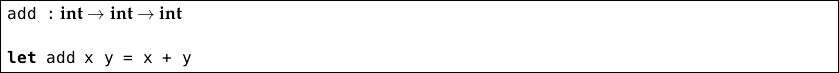We have been considering functions like this as taking two arguments and returning a result. In fact, the truth is a little different. The type int int int can also be written as int (int int). OCaml lets us omit the parentheses because is a right-associative operator in the language of types. This gives us a clue.

In truth, the function `add` is a function which, when you give it an integer, gives you a function which, when you give it an integer, gives the sum.

This would be of no particular interest to us, except for one thing: we can give a function with two arguments just one argument at a time, and it turns out to be rather useful. For example:

` OCaml`

`# let add x y = x + y`
`val add : int -> int -> int = <fun>`
`# let f = add 6`
`val f : int -> int = <fun>`
`# f 5`
`- : int = 11`

Here, we have defined a function `f` by applying just one argument to `add`. This gives a function of type int int which adds six to any number. We then apply `5` to this function, giving `11`. When defining `f`, we used partial application (we applied only some of the arguments). In fact, even when applying all the arguments at once, we could equally write `(add 6) 5` rather than `add 6 5`. We can add six to every element in a list:

`map (add 6) [10; 20; 30]`

Here, `add 6` has the type int int, which is an appropriate type to be the first argument to `map` when mapping over a list of integers. We can use partial application to simplify some examples from earlier in the book. We mentioned that you can write, for example, `( * )` to produce a function from an operator. It has type int int int. We may partially apply this function, so instead of writing

`map (fun x -> x * 2) [10; 20; 30]`

we may write

`map (( * ) 2) [10; 20; 30]`

Recall the function to map something over a list of lists from the questions to Chapter 6: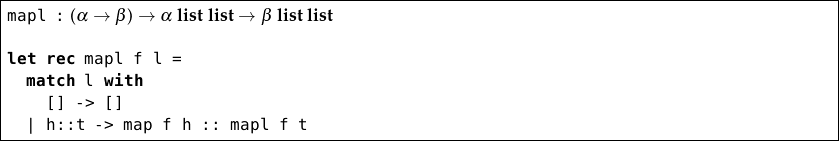With partial application, we can write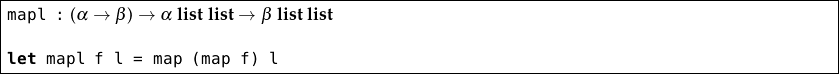Can you see why? The partially applied function `map f` is of type α list β list, which is exactly the right type to pass to `map` when mapping over lists of lists. In fact, we can go even further and write: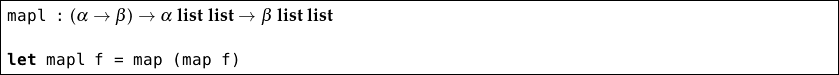Here,  `map (map f)` has type α list list β list list so when an `f` is supplied to `mapl`, a function is returned requiring just the list. This is partial application at work again.

You can see the real structure of multiple-argument functions, by writing `add` using anonymous functions: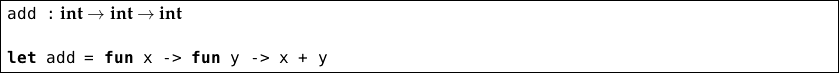This makes it more obvious that our two-argument `add` function is really just composed of one-argument functions, but `let add x y = x + y` is much clearer! We can apply one or more arguments at a time, but they must be applied in order. Everything in this chapter also works for functions with more than two arguments.

## Summary

The function `f x y` has type α β γ which can also be written α (β γ). Thus, it takes an argument of type α and returns a function of type β γ which, when you give it an argument of type β returns something of type γ. And so, we can apply just one argument to the function `f` (which is called partial application), or apply both at once. When we write `let`` f x y = …` this is just shorthand for `let`` f = ``fun`` x -> ``fun`` y -> …`

## Questions

1. Rewrite the summary paragraph at the end of this chapter for the three argument function `g a b c`.

2. Recall the function `member x l` which determines if an element `x` is contained in a list `l`. What is its type? What is the type of `member x`? Use partial application to write a function `member_all x ls` which determines if an element is a member of all the lists in the list of lists `ls`.

3. Why can we not write a function to halve all the elements of a list like this: `map (( / ) 2) [10; 20; 30]`? Write a suitable division function which can be partially applied in the manner we require.

4. Write a function `mapll` which maps a function over lists of lists of lists. You must not use the `let rec` construct. Is it possible to write a function which works like `map`, `mapl`, or `mapll` depending upon the list given to it?

5. Write a function `truncate` which takes an integer and a list of lists, and returns a list of lists, each of which has been truncated to the given length. If a list is shorter than the given length, it is unchanged. Make use of partial application.

6. Write a function which takes a list of lists of integers and returns the list composed of all the first elements of the lists. If a list is empty, a given number should be used in place of its first element.